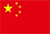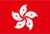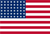• 下载App

扫码立即下载

+

• 首页
• 行情
• 资讯
• 牛牛圈
• 课堂
• 帮助中心
• 关于
• 语言
•••EN

# A Look At The Intrinsic Value Of Lindsay Corporation (NYSE:LNN)

Simply Wall St ·  03/14 00:03

### Key Insights

• Using the 2 Stage Free Cash Flow to Equity, Lindsay fair value estimate is US\$125
• Lindsay's US\$144 share price indicates it is trading at similar levels as its fair value estimate
• Analyst price target for LNN is US\$187, which is 49% above our fair value estimate

Does the March share price for Lindsay Corporation (NYSE:LNN) reflect what it's really worth? Today, we will estimate the stock's intrinsic value by taking the forecast future cash flows of the company and discounting them back to today's value. One way to achieve this is by employing the Discounted Cash Flow (DCF) model. Don't get put off by the jargon, the math behind it is actually quite straightforward.

We generally believe that a company's value is the present value of all of the cash it will generate in the future. However, a DCF is just one valuation metric among many, and it is not without flaws. For those who are keen learners of equity analysis, the Simply Wall St analysis model here may be something of interest to you.

See our latest analysis for Lindsay

## The Method

We are going to use a two-stage DCF model, which, as the name states, takes into account two stages of growth. The first stage is generally a higher growth period which levels off heading towards the terminal value, captured in the second 'steady growth' period. To begin with, we have to get estimates of the next ten years of cash flows. Where possible we use analyst estimates, but when these aren't available we extrapolate the previous free cash flow (FCF) from the last estimate or reported value. We assume companies with shrinking free cash flow will slow their rate of shrinkage, and that companies with growing free cash flow will see their growth rate slow, over this period. We do this to reflect that growth tends to slow more in the early years than it does in later years.

Generally we assume that a dollar today is more valuable than a dollar in the future, and so the sum of these future cash flows is then discounted to today's value:

#### 10-year free cash flow (FCF) estimate

 2023 2024 2025 2026 2027 2028 2029 2030 2031 2032 Levered FCF (\$, Millions) US\$89.2m US\$92.9m US\$85.6m US\$92.2m US\$92.9m US\$94.0m US\$95.3m US\$96.8m US\$98.5m US\$100.4m Growth Rate Estimate Source Analyst x1 Analyst x1 Analyst x1 Analyst x1 Est @ 0.75% Est @ 1.15% Est @ 1.42% Est @ 1.62% Est @ 1.75% Est @ 1.85% Present Value (\$, Millions) Discounted @ 8.2% US\$82.4 US\$79.4 US\$67.6 US\$67.3 US\$62.6 US\$58.6 US\$54.9 US\$51.6 US\$48.5 US\$45.6

("Est" = FCF growth rate estimated by Simply Wall St)
Present Value of 10-year Cash Flow (PVCF) = US\$618m

After calculating the present value of future cash flows in the initial 10-year period, we need to calculate the Terminal Value, which accounts for all future cash flows beyond the first stage. For a number of reasons a very conservative growth rate is used that cannot exceed that of a country's GDP growth. In this case we have used the 5-year average of the 10-year government bond yield (2.1%) to estimate future growth. In the same way as with the 10-year 'growth' period, we discount future cash flows to today's value, using a cost of equity of 8.2%.

Terminal Value (TV)= FCF2032 × (1 + g) ÷ (r – g) = US\$100m× (1 + 2.1%) ÷ (8.2%– 2.1%) = US\$1.7b

Present Value of Terminal Value (PVTV)= TV / (1 + r)10= US\$1.7b÷ ( 1 + 8.2%)10= US\$760m

The total value, or equity value, is then the sum of the present value of the future cash flows, which in this case is US\$1.4b. In the final step we divide the equity value by the number of shares outstanding. Compared to the current share price of US\$144, the company appears around fair value at the time of writing. Remember though, that this is just an approximate valuation, and like any complex formula - garbage in, garbage out.

## Important Assumptions

The calculation above is very dependent on two assumptions. The first is the discount rate and the other is the cash flows. You don't have to agree with these inputs, I recommend redoing the calculations yourself and playing with them. The DCF also does not consider the possible cyclicality of an industry, or a company's future capital requirements, so it does not give a full picture of a company's potential performance. Given that we are looking at Lindsay as potential shareholders, the cost of equity is used as the discount rate, rather than the cost of capital (or weighted average cost of capital, WACC) which accounts for debt. In this calculation we've used 8.2%, which is based on a levered beta of 1.032. Beta is a measure of a stock's volatility, compared to the market as a whole. We get our beta from the industry average beta of globally comparable companies, with an imposed limit between 0.8 and 2.0, which is a reasonable range for a stable business.

### SWOT Analysis for Lindsay

Strength
• Earnings growth over the past year exceeded the industry.
• Debt is not viewed as a risk.
• Balance sheet summary for LNN.
Weakness
• Dividend is low compared to the top 25% of dividend payers in the Machinery market.
• Expensive based on P/E ratio and estimated fair value.
Opportunity
• Annual earnings are forecast to grow for the next 3 years.
Threat
• Dividends are not covered by cash flow.
• Annual earnings are forecast to grow slower than the American market.
• See LNN's dividend history.